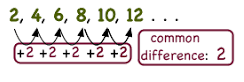An Arithmetic Progression is a sequence of numbers in which we get each term by adding a particular number to the previous term, except the first term.• Each number in the sequence is known as term.
• The fixed number i.e. the difference between each term with its preceding term is known as common difference. It can be positive, negative or zero. It is represented as ‘d’.
Some Examples of Arithmetic Progressions
Common difference Value of d Example
d > 0, positive 10 20, 30, 40, 50,…
d < 0, negative -25 100, 75, 50, 25, 0
d = 0, zero 0 5, 5, 5, 5,..
General form of Arithmetic Progression

Where the first term is ‘a’ and the common difference is ‘d’.